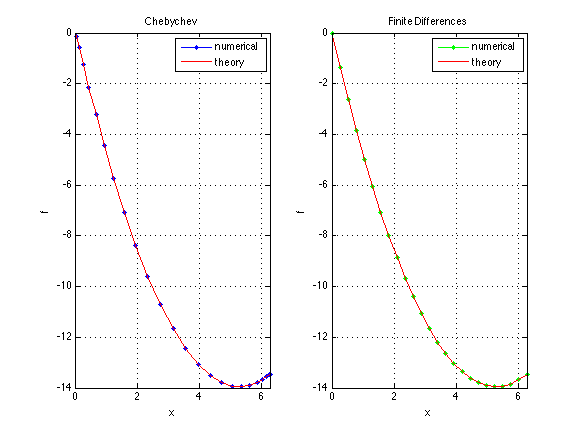# Solving a linear differential equation with Chebychev differentiation matrix

This code is built upon diffmat.m and differential_equation.m where the differentiation matrices are built. Here we solve a linear non-homogeneous differential equation in 1D with non-homogeneous boundary conditions with the finite differences way and with the function based on Chebychev.

We show here how to use the differentiation matrix to code the differential equation as a linear system and impose Dirichlet and Neuman boundaryconditions.

    clear all; clf

% parameters
L=2*pi; % domain length
N=25; % number of points

% the grid
y=linspace(0,L,N)';
h=y(2)-y(1); % the grid size

%% first derivative
D=zeros(N,N);
D(1,1:3)=[-3/2, 2, -1/2]/h;
for ind=2:N-1
D(ind,ind-1:ind+1)=[-1/2, 0, 1/2]/h;
end
D(end,end-2:end)=[1/2, -2, 3/2]/h;

% second derivative
DD=zeros(N,N);
DD(1,1:3)=[1, -2, 1]/h^2;
for ind=2:N-1
DD(ind,ind-1:ind+1)=[1, -2, 1]/h^2;
end
DD(end,end-2:end)=[1, -2, 1]/h^2;


In this part, we use the chebdif() function (that we can find here chebdif.m) to calculate the first and seconde derivative of the function.

    %% differentiation matrices
scale=-2/L;
[x,DM] = chebdif(N,2);
dx=DM(:,:,1)*scale;
dxx=DM(:,:,2)*scale^2;
x=(x-1)/scale;
Z=zeros(N,N); I=eye(N);

%% I build the linear differential equation
A=dxx;
B=DD;

%% boundary conditions
A([1,N],:)=[I(1,:); dx(N,:)];
B([1,N],:)=[I(1,:); D(N,:)];
b=1+zeros(N,1); b([1,N])=[0,1];

%% solve the system
f=A\b;
g=B\b;

%% plotting the Chebychev differentiation way
subplot(1,2,1)
plot(x,f,'b.-',x,x.^2/2+(1-L)*x,'r-');
xlabel('x');ylabel('f');
legend('numerical','theory')
xlim([0,L]); grid on
title('Chebychev');

%% plotting the finite differentiation way
subplot(1,2,2)
plot(y,g,'g.-',y,y.^2/2+(1-L)*y,'r-');
xlabel('x');ylabel('f');
legend('numerical','theory')
xlim([0,L]); grid on
title('Finite Differences');

set(gcf,'paperpositionmode','auto')
print('-dpng','-r75','differential_equation_cheb.png')%}